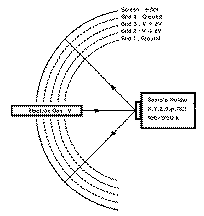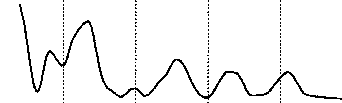## Data Format

Most of the files are in the Stony Brook/IBM format.
Each beam (curve) has one header line: Fortran '(80A1)' or '(A80)'
Each data line has six pairs of energy/intensity: Fortran '(12F6.2)'
Sometimes each data-line has a beam `ID #' in columns 73-76. Each beam ends with a '5' in column 80: Fortran '(79X,A1)'
Each files ends with a 'stop': Fortran '(A4)'
Each beam usually has its maximum intensity scaled to 100.00. Hence, all intensities are relative.

This format goes back to the days of of punch tape and punch cards. The format is portable and arcane, so please no comments.

Some data might exist after the stop; usually it is extra beams, or data that was truncated for plotting purposes.

```      Subroutine REEXBM(XEX,YEX,MaxPt,NUMPTS,NDONE)
c       ReExBm will read in data from the experimental data file.
c       XeX : is the input Energy Vector
c       YeX : is the input Intensity Vector
c       MaxPt : is the dimensioned size of the above vectors
c       NumPts : is the number of data points used in the vectors
c       NDone : is a flag set to 1 when the file is finished.
Parameter(MCC=20)
Dimension XEX(MaxPt),YEX(MaxPt),FMT(MCC)
CHARACTER*4 FMT,NULL
NULL='stop'
NDONE=0
IF( Fmt( 1 ) .Ne. Null ) Then
IR = -5
ID = 0
Irp5 = 0
DO WHILE(ID .NE. 5)
IR = IR + 6
IRP5 = IR + 5
1                 (XEX(I),YEX(I),I=IR,IRP5),NC,ID
EndDo ! DO WHILE
NUMPTS=IRP5
DO WHILE (XEX(NUMPTS).EQ.0.0.OR.YEX(NUMPTS).EQ.0.0)
NUMPTS=NUMPTS-1
EndDo ! of do while
I = 1
DO WHILE ( XEX(I) .lt. 0.0000001 )
I = I + 1
EndDo !DO WHILE
IF( I .NE. 1 ) THEN
NUMPTS = NUMPTS - I + 1
J = I - 1
DO IG = 1 , NUMPTS
XEX( ig ) = XEX( ig + J )
YEX( ig ) = YEX( ig + J )
EndDo !IG
EndIf ! IF I<>1
NDONE = 1
EndIf ! DIDN'T READ A 'stop'
Return
END  !^^^^^^^^^^^^^^^^^^^^^^^^^^^^^^^^^^^^^^^^^^^^^^^^^^^^^^^^
c     ^^^^^^^^^^^^^^^^^^^^^^^^^^^^^^^^^^^^^^^^^^^^^^^^^^^^^^^^^^^^^^
```

There is no guarantee of accuracy given with this data.